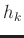# Appendix A: Local Similarity

In comparing two datasets, the purpose is to estimate a smoothly varying warping function,, required to align one dataset,, to a reference dataset,,(19)

We can represent the warping function with the shifts,, as follows:(20)

where thedenotes the original independent axis andare the shifts required to match the datasets as defined in Equation A-1. The correlation coefficient can be used to quantify the quality of the match between datasets (Hampson-Russell, 1999). The LSIM method begins with the observation that the correlation coefficient () only provides one number to describe the datasets; however, we am interested in understanding the local changes in the datasets' similarity. Therefore, the LSIM method computes local similarity, which is a function of time,. The square ofcan be split into a product of two factors (Fomel, 2007a):(21)

whereandare the solutions to the following regularized least-squares problems, respectivelyThe regularization operator,, is implemented using shaping regularization (Fomel, 2007b) and designed to enforce smoothness. To estimate the solution, LSIM is calculated for a series of shifts. The results of this calculation are accumulated and displayed on a `similarity scan.'

2019-05-07